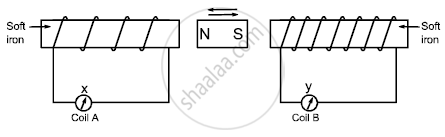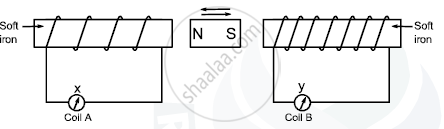# A Magnet Kept at the Centre of Two Coils a and B is Moved to and Fro as Shown in the Diagram.A Magnet Kept at the Centre of Two Coils a and B is Moved to and Fro as Shown in the Diagram. - Physics

Short Note

A magnet kept at the centre of two coils A and B is moved to and fro as shown in the diagram. The two galvanometers show deflection.State with a reason whether :

x > y

or

x< y    [x and y are magnitudes of deflection.]

#### Solution∴ The no. of turns in B is greatet than A
∴ Deflection in B will be greater then deflection in A

y > x

Concept: Effects of Electric Current - Magnetic Effect of Electric Current
Is there an error in this question or solution?